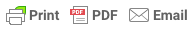Sorry, you do not have permission to ask a question, You must login to ask question. Become VIP Member

Measurement of reinforced concrete works such as foundation, columns, beams, slabs pedestal are needed for calculation of actual cost of construction and payment to the contractor.

This article provides guidelines for the measurement including formulas for the calculation of quantities of various reinforced concrete works.

## Measurement of Reinforced Concrete Works:

1. The Quantities can be rounded off to the nearest two decimals.
2. Concrete in Structural members, such as columns, Beams and slabs shall each be measured separately.
3. No Deductions shall be made for the following:
• Opening up to 0.1 m2
• Volume occupied by reinforcement.
• Volume occupied by pipes, conduits, sheathing, etc not exceeding 100cm2 each in cross-section.
• Moulds, drips moulding, chamfers, splays rounded or covered angles, beds, grooves and rebates up to 10 cm in girth.

## Measurement of Concrete in Footings:

Volume of concrete = L x B x D for Rectangular and Square footing. For Trapezoidal Footing, Volume of Concrete is calculated in two parts. Bottom Rectangular portion is calculated separately and Trapezoidal volume is calculated separately.

Rectangular Volume = L x B x D

Trapezoidal Volume V= H/3 (A1 + A2 +  SQRT(A1 + A2) )

Where A1 and A2 is the area of top and bottom rectangles, H is depth of footing.

Total Volume = Rectangular Volume + Trapezoidal Volume

Where V= Volume; h= height;& Ad Free!

GET VIP MEMBERSHIP NOW!

## Measurement of Concrete in Columns

Columns shall be measured from top of Column base to underside of first floor slab and subsequently from top of floor slab to underside of floor slab above. In case of Columns for flat slabs, flare of column shall be included with column for Measurement.

## Measurement of Concrete in Beams

Beams shall be measured from face to face of columns and shall include haunches, if any, between columns and beams. The depth of beams shall be measured from bottom of slab to bottom of the beam except in case of inverted beam where it shall be measured from top of slab to top of beam.## Measurement of Concrete in Pedestals

Pedestals is measured from top of Footing to top/ Bottom of plinth beam depending on site conditions. If measured up to bottom of Plinth beam then Ground floor column can be measured from bottom of plinth beam to underside of slab.

The Height of Pedestal can be arrived from levels of footing and plinth beam. The Height shall be crosschecked with site also.

#### Gopal Mishra

Gopal Mishra is a Civil Engineer from NIT Calicut and has more than 10 years of experience in Civil Engineering and Construction. He is the founder of The Constructor.

## How to Deal With a Wet Basement?

1. (L+Y/2) x H x M … try this formula for trapezoidal beam
.
(note )
L=Top of beam
Y=Bottom of beam

• Share
2. (L+Y/2) x H x M … try this formula for trapezoidal beam
.
(note )
L=Top of beam
Y=Bottom of beam

• Share
3. (L+Y/2) x H x M …can u try this formula for trapezoidal beam cuz its better than ur way
.
(note :)
L=Top of beam
Y=Bottom of beam

• Share
4.on which basis we r going with the formula of trapazoidal in footing
Trapezoidal Volume V= H/3 (A1 + A2 + SQRT(A1 + A2) )
. . is der any proper IS CODE or specifications dat we can allow dis much quantity because with my reference i vll get
Trapezoidal Volume V= H/3 (A1 + A2 + SQRT(A1 *A2) )
dos formula for trap section. .

• Share
5. Kndly correct the volumetric formulae of trapezoidal part………kamal nayan

• Share
7.